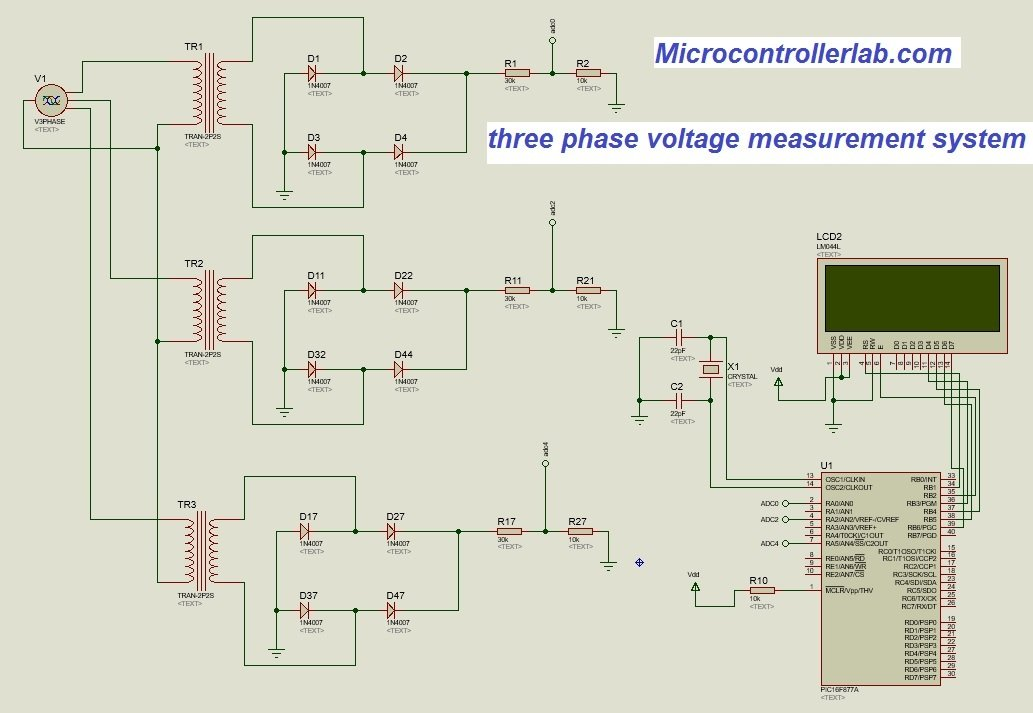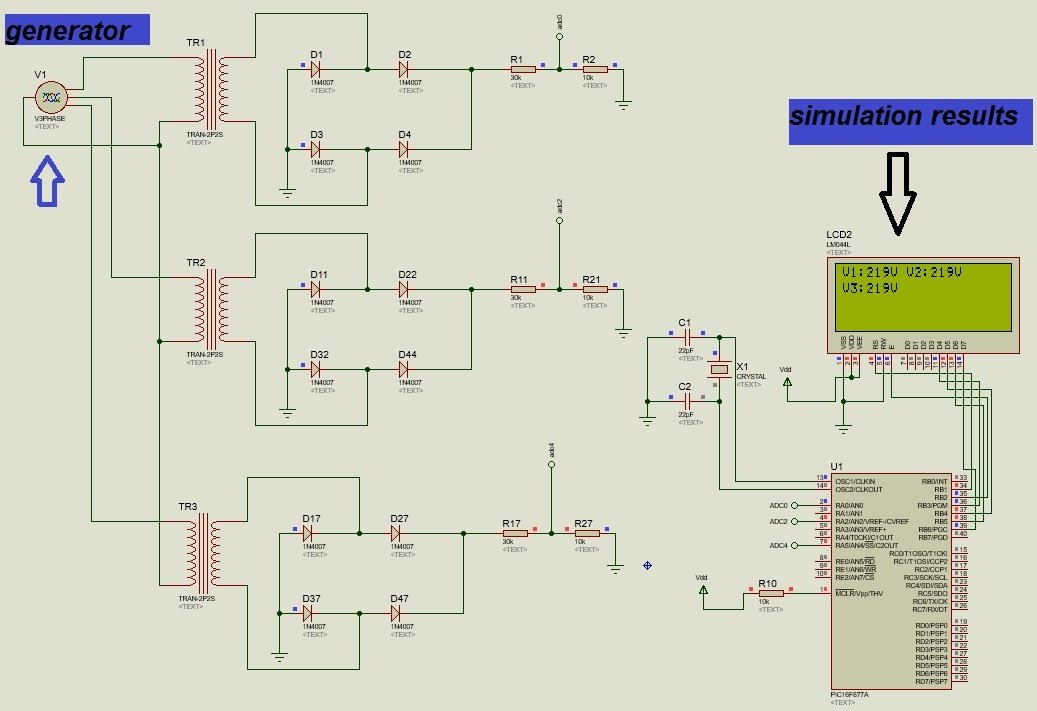# Three phase voltage measurement using pic microcontroller

Three-phase voltage measurement using pic microcontroller project: Hi everyone I hope you are fine and doing well. In this tutorial, you will learn about how to measure three phase voltage using pic microcontroller. Three-phase voltage measurement has many applications in power as well as the electronics field. Three-phase voltage measurement system is used to measure the voltage of three-phase transmission lines, three-phase generator, three-phase transformer, three-phase induction motor, and any other three-phase device.

## Where to use Three Phase Voltage Measurement System?

This project can be used for the protection of these devices from over and under-voltage. Over and under-voltage protection systems are designed to protect these devices for damaging under over and under-voltage conditions just like a circuit breaker. But circuit breaker usually works on the mechanical phenomenon and in this digital world, every electrical project or electronic project becomes more and more digital. So protection devices are also using digital intelligent circuit breakers. In these devices, microcontrollers are used to sense voltage and current of every phase. So this three-phase voltage measurement system is useful for solid-state electronic relays.

## Necessary Background

Before we start our discussion on three phase voltage measurement system, you should know how to use a pic microcontroller for ac voltage measurement. I  recommend you to read this ac voltage measurement using pic microcontroller article before going further. In this article, I have discussed in detail how to measure peak voltage of sine wave and how to use ADC of pic microcontroller to measure sinusoidal voltage which is changing with time.

## Required Components

Following is a list of component used in three phase voltage measurement system:

### Step Down Transformer

Step down transformer is used to step down voltage from 220 volts to 12 volts. Because we can not measure higher voltage directly with a pic microcontroller or any microcontroller. We can also use a voltage limiter circuit using a resistive network. But that will cause power dissipation issues.

### Full-wave Rectifier

After step down transformer, a full-wave rectifier is used. A full-wave rectifier is used to convert a negative cycle of ac voltage to a positive cycle. Because we can also not measure negative voltage directly with a microcontroller. Because reference voltage for a controller is ground which is zero volt actually. So it is necessary to convert a negative half cycle into a positive cycle. I assume you are already familiar with working of full wave rectification. If not, I recommend you to read any secondary level physics book.

### Voltage Divider Circuit

After full wave rectification, voltage divider circuit is used. The voltage we get at the output of the full-wave rectifier is around 17-volt dc and 12 volt RMS voltage. Again this voltage is more than a range of pic16f877a microcontroller. Pic16f877a operating voltages range is between 0 and 5 volt. So maximum voltage which we can measure with the controller is 5 volt and the minimum is 0 volt. So we need to used voltage divider circuit to step down voltage further.

### PIC microcontroller

After the voltage divider circuit, the Pic16f877a controller is used. Output of voltage divider is connected with analog channels of a controller which can read analog voltage and convert it into digital value. This measured digital value is used to calculate actual ac voltage with the help of mathematical calculation in programming.

### 20×4 LCD display

The liquid crystal display displays the values of voltage for each phase. We can easily interface LCD with any pic microcontroller using GPIO ports. You can read this complete tutorial on LCD interfacing.

LCD Interfacing with PIC Microcontroller

## 3 Phase Voltage Measurement Circuit Diagram

The circuit diagram of three-phase voltage measurement system is given below. As you can see in the circuit diagram, we are using three phase generator and the voltage of each phase is measuring with the same circuit. Phase one, phase two and phase thee measurement circuit are the same. Therefore, the working for all phase remains the same as I discussed above for every component. In this circuit diagram, we used labels to make connection of output circuits with a microcontroller. So make sure to make an actual connection when you design this circuit.## Simulation Result in Proteus

The simulation results of three-phase voltage measurement system are shown below. V1 shows a voltage of phase one, V2 shows a voltage of phase 2 and similarly v3 shows a voltage of phase 3.### Code of three-phase voltage measurement using pic microcontroller

It is very easy to write code for this system. you should know how to use analog to digital converter of pic microcontroller and how to interface LCD with pic microcontroller. Other than this you just need simple c programming concepts. To measure ac voltage, we have divided each positive cycle into 400 steps. With 5oHZ frequency time of each positive half, the cycle is 10ms. So we are talking 400 samples in 10ms to get maximum voltage or peak voltage of sine wave. The maximum value finding algorithm is used to find maximum value from these 400 samples. After finding the maximum sample, the digital value of this sample is converted back into a voltage by multiplying in with the resolution factor of pic16f877a ADC.  After that voltage divider and transformer turns ratio is used to get actual voltage. This actual voltage is displayed on LCD. Contact us to get code.## Other Pic Microcontroller Projects

### 10 thoughts on “Three phase voltage measurement using pic microcontroller”

1. Hello Sir, can I get the code of PIC of this project ?

2. how to measure and record three phase power

3. hello sir,, how do you measure v line- line ?

4. Wow. This asshole wants money for the code. What an ass.

5. Código é feito em Assembly?

6. Hello!
Thanks for the good works you have been publishing here.
can you develop the arduino version of the three phase AC measurement capable of reading the inputs from the 3 different ADC pins, converting them into actual AC voltage equivalents and displaying them on the LCD. I want to study and buy the design and its code.

Thank you in anticipation of your response.